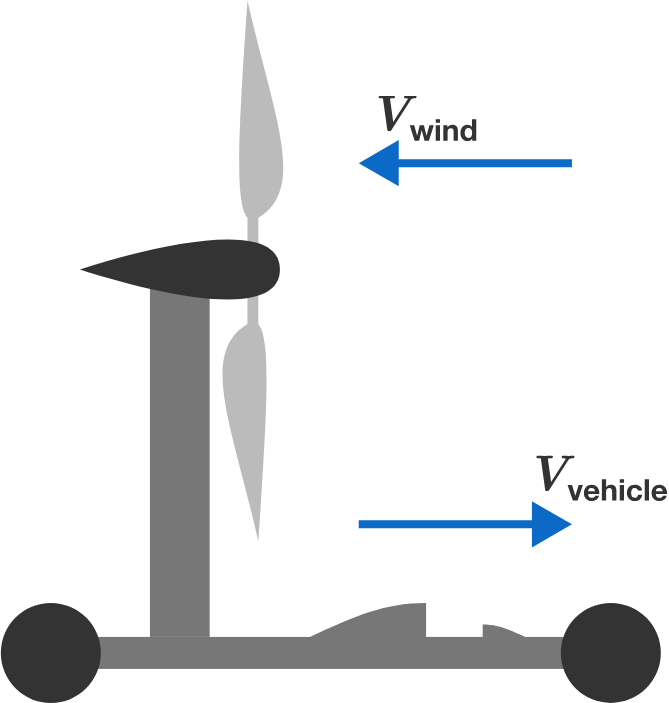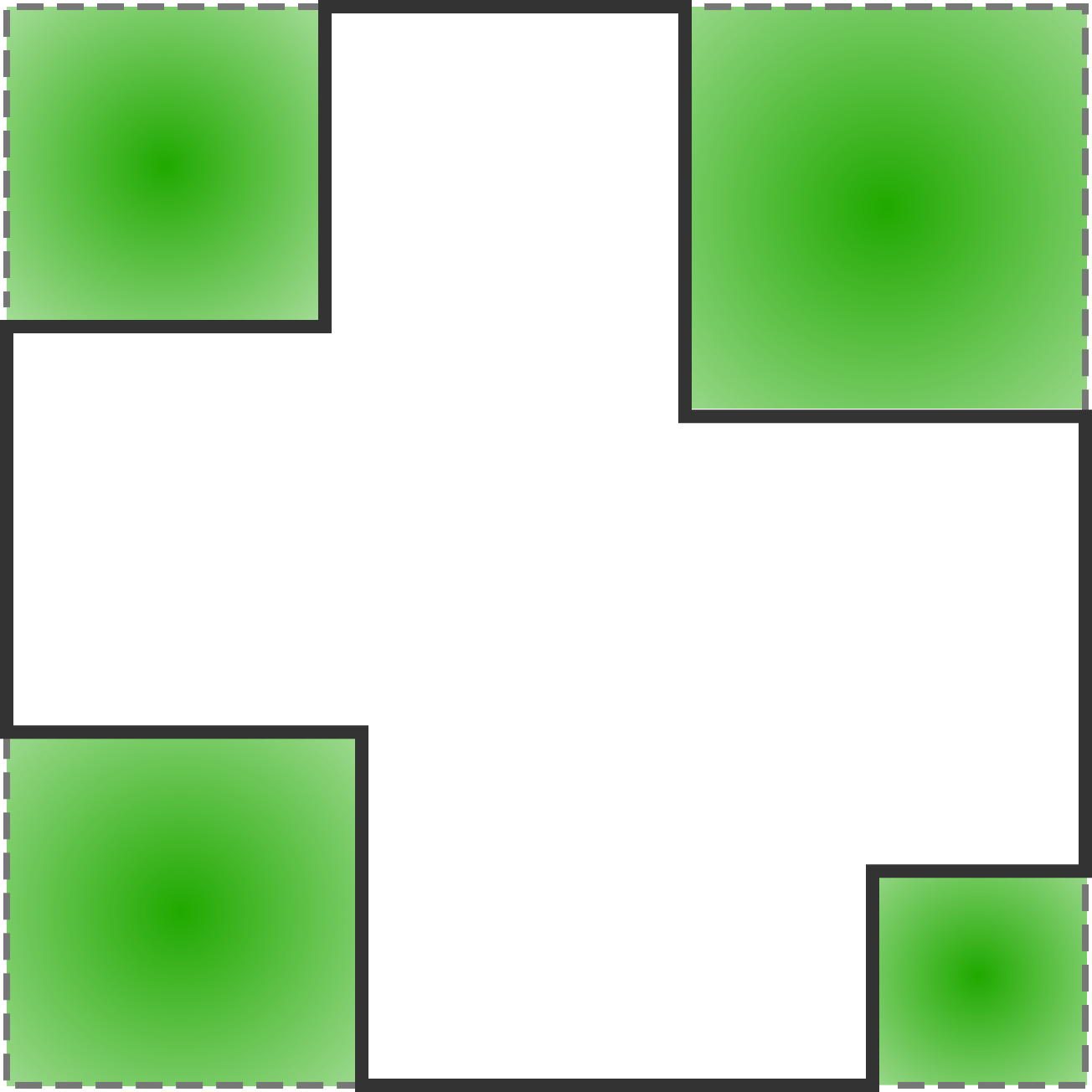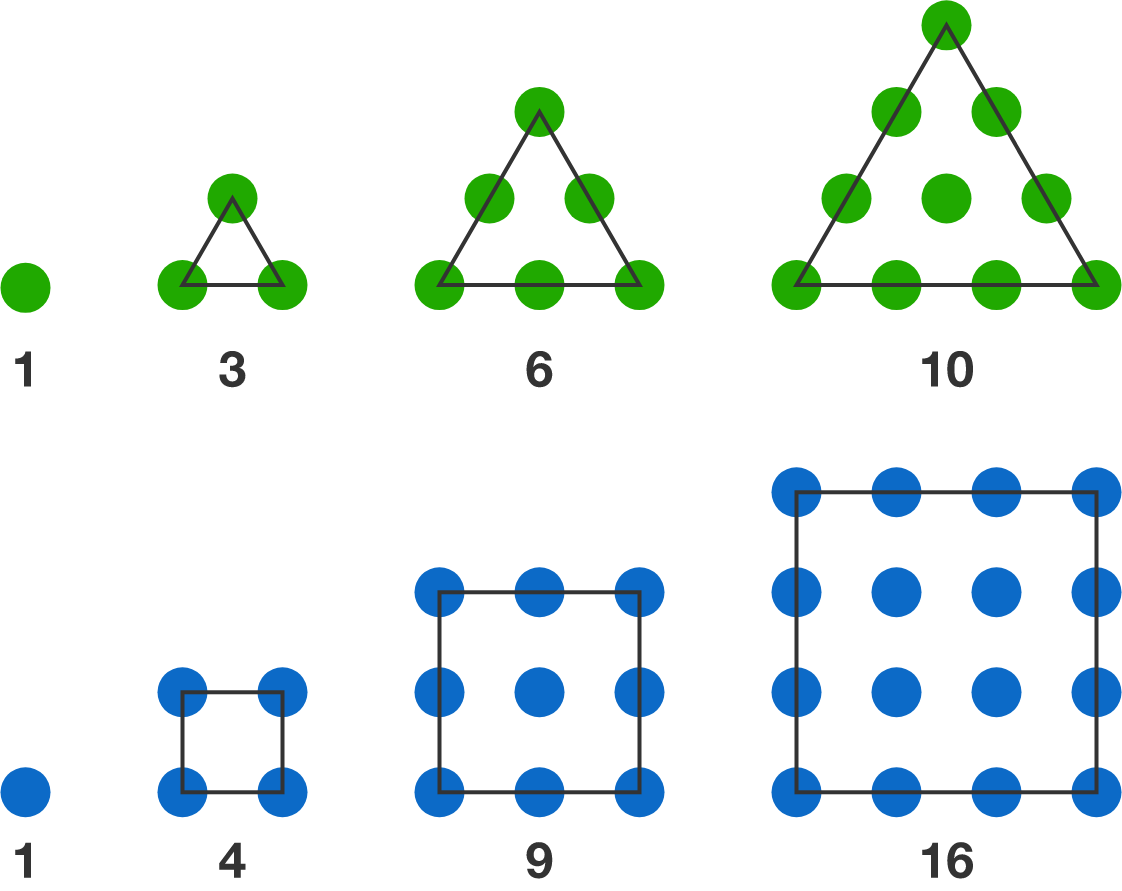# Problems of the Week

Contribute a problem

# 2018-10-29 BasicCan you fill in the blank?

Consider the plan below, which consists of a wheeled vehicle and a wind turbine that's connected to the wheels by nothing but a mechanical gear (there's no engine aboard). The inventor claims that it's a vehicle that can travel upwind, powered by the wind itself, and that it doesn't move if there's no wind.

Does this proposal violate either of the following principles and, if so, which one?My house (in white) and four square gardens (in green) are built on a square land of $400\text{ m}^2.$

What is the perimeter of my house?

True or False?

The sum of two consecutive triangular numbers is always a square number.The first four triangular numbers and the first four square numbers.

$\begin{cases} x + y = \dfrac xy \\\\\\\\ x - y = xy \end{cases}$

Are there any real solutions satisfying this system of equations?

×